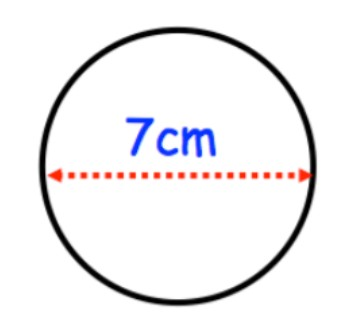In the space, draw a circle of diameter 7 cm.Verified
148.5k+ views
Hint:In math, a circle is defined as the set of points on a plane (a flat surface that is infinite in every direction) that are all the same distance from a given point. The given point is called the center of the circle. The same distance is called the radius. In circle construction, the center is where you put the metal point of the compass, the radius is how wide you set the two arms of the compass, and the circle is the curved line that you draw.

Here are the steps for drawing a perfect circle:
1) Locate and mark the place where you want the center of your circle.

2) Determine the radius. It may be a measurement or a distance between other points on the drawing. Make sure you know exactly how long the radius should be.In the question they given diameter of circle so we know relation between diameter and radius i.e $\text{Radius}=\dfrac{\text{Diameter}}{2}=\dfrac{7}{2}=3.5$
Hence the radius = 3.5 cm

3) Put the compass point and pencil lead close to each other, and make sure they are set to roughly the same length. That will make the circle easier to draw. Tighten the grips on the pencil and compass point.

4) Widen the arms of the compass to the radius you want, and tighten the hinge at the top (as in lock the arms).

5) Re-measure your radius, just to make sure the compass arms did not slip.

6) Place the metal point of the compass on the mark you made for the center of the circle.Note:Pressing evenly on the metal point and pencil, turn the knob at the top of the compass, moving the pencil point all the way around the circle. If the circle is too light, do it again, pressing a little harder.